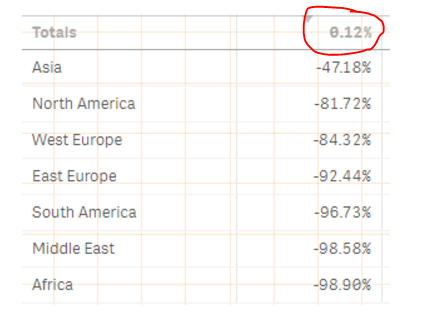New to QlikView

Discussion board where members can get started with QlikView.

Announcements
Highlighted
New Contributor III

Calculating percentage for each row in a table

Hi everyone,

I'm trying to figure out how to calculate the percentage (=((Sum([CY 2017]) - Sum([Prev.CY 2017])) / Sum([Prev.CY 2017]))) for each row in the table accuractely. Here's a picture for reference.As you can see, the numbers aren't accurate. Please help.

Tags (2)
10 RepliesMVP

Re: Calculating percentage for each row in a table

 As you can see, the numbers aren't accurate. Please help.

I am not sure I see the inaccurate numbers? What are you expecting to see? How does the raw data looks like?

Valued Contributor III

Re: Calculating percentage for each row in a table

do you complain about the total value?

New Contributor III

Re: Calculating percentage for each row in a table

I don't understand...MVP

Re: Calculating percentage for each row in a table

Robin wants to know if you are unhappy with this numberValued Contributor III

Re: Calculating percentage for each row in a table

me too ...  what result do you expect?

Just post a sample please and explain what you're expecting.

New Contributor III

Re: Calculating percentage for each row in a table

The percentages are too high. They should be much lower than what they are...MVP

Re: Calculating percentage for each row in a table

May be check if individual part of the expressions make sense

1) Sum([CY 2017])

2) Sum([Prev.CY 2017])

Can you share a screenshot with Region as dimension and the above two as expressions?

New Contributor III

Re: Calculating percentage for each row in a table

The total is correct. It's the regions that are not...

New Contributor III

Re: Calculating percentage for each row in a table

I'm expecting the variances for Asia, as an example, to be 0.1% for 2017, 0.0% for 2018, 0.1% for 2019, 0.2% for 2020, 0.6% for 2021, and 0.6% for 2022.